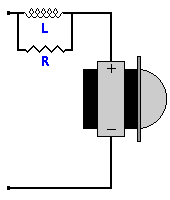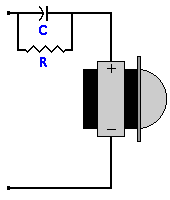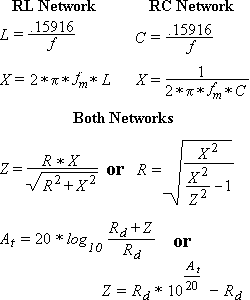# DIY Audio & Video

## Contour Network Designer / Calculator Help

Use the Contour Network Designer.

A contour network is part of the crossover that is used to correct a rising frequency response from a driver in your speaker. There are two types of contour networks. The first is a RL (Resistor & Inductor) network that is used when the response is rising as the frequency increases. The other network is a RC (Resistor & Capacitor) network for drivers whose response is rising as the frequency decreases.

The contour network formulas are not exact. They should only be used as starting point for designing a contour network. With the proper test equipment and trial and error you will be able to find the right component values for your network.

The following formulas are for both types of networks. In some cases, different derivations of the same formula are given. In the reading material I have available, the formulas provided were to find Z and At given R, X, & Rd. From this, I created the formulas to find Z and R given At, Rd, & X.f = Frequency where response starts in increase in Hz

fm = Frequency where response is at its peak in Hz

At = Attenuation, increase in db from f to fm

Rd = Total Driver Impedance including all circuits in Ohms

X = Component (L or C) reactance at fm

Z = Total circuit impedance in Ohms